# 700 Pick Your Pony! Who will win this Amount of Factors Horse Race?

• 700 is a composite number.
• Prime factorization: 700 = 2 x 2 x 5 x 5 x 7, which can be written 700 = (2^2) x (5^2) x 7
• The exponents in the prime factorization are 2, 2 and 1. Adding one to each and multiplying we get (2 + 1)(2 + 1)(1 + 1) = 3 x 3 x 2 = 18. Therefore 700 has exactly 18 factors.
• Factors of 700: 1, 2, 4, 5, 7, 10, 14, 20, 25, 28, 35, 50, 70, 100, 140, 175, 350, 700
• Factor pairs: 700 = 1 x 700, 2 x 350, 4 x 175, 5 x 140, 7 x 100, 10 x 70, 14 x 50, 20 x 35 or 25 x 28
• Taking the factor pair with the largest square number factor, we get √700 = (√100)(√7) = 10√7 ≈ 26.457513.Because this is my 700th post, I think I’ll have another horse race. Some numbers from 601 to 700 have exactly 2 factors, 4 factors, and so forth up to 24 factors. (Only perfect squares can have an odd number of factors.)

Which number from 1 to 24 will win this amount of factors horse race? Which number will come in second place, or third place? Cheering for more than one pony will make the race even more interesting.

Here we see that the numbers 2, 6, & 8 are the first ones out of the gate. Click on the graphic to see the rest of this very thrilling horse race:Every hundred posts I also like to focus on the percentage of numbers whose square roots can be simplified.

700 is divisible by 100 so its square root can easily be simplified: √700 = 10√7.

273 of the first 700 numbers have reducible square roots. That’s exactly 39%.

The rest of the numbers, 427, which is 41% of the first 700 numbers, do not have reducible square roots.

Here’s a table breaking down the amount of factors in each group of one hundred integers and the number of reducible square roots.Here are some facts about the number 700.

700 is a palindrome in several bases:

• 4A4 BASE 12; note A is equivalent to 1o in base 10, and 4(144) + 10(12) + 4(1) = 700
• PP BASE 27; note P is equivalent to 25 in base 10, and 25(27) + 25(1) = 700
• KK Base 34; note K is equivalent to 20 in base 10, and 20(34) + 20(1) = 700

700 is the sum of four consecutive prime numbers: 167 + 173 + 179 + 181.

Here is a beautiful painting of a horse race that I saw on twitter:

# 508 A Mathematical and Biblical Truth: 2 > 1Two are better than one; because they have a good reward for their labour.

For if they fall, the one will lift up his fellow: but woe to him that is alone when he falleth; for he hath not another to help him up.

Ecclesiastes 4: 9 – 10

—————————————————————————————————

508 is the sum of some consecutive prime numbers, and at least one of those prime numbers is in its prime factorization. Can you figure out what those consecutive primes are?

• 508 is a composite number.
• Prime factorization: 508 = 2 x 2 x 127, which can be written 508 = (2^2) x 127
• The exponents in the prime factorization are 2 and 1. Adding one to each and multiplying we get (2 + 1)(1 + 1) = 3 x 2  = 6. Therefore 508 has exactly 6 factors.
• Factors of 508: 1, 2, 4, 127, 254, 508
• Factor pairs: 508 = 1 x 508, 2 x 254, or 4 x 127
• Taking the factor pair with the largest square number factor, we get √508 = (√4)(√127) = 2√127 ≈ 22.53 88 55 33 9# 336 and Level 2

### Today’s Puzzle:

The sixteen clues given in this puzzle are all you need to complete this multiplication table!Print the puzzles or type the factors on this excel file: 12 Factors 2014-12-29### Factors of 336:

• 336 is a composite number.
• Prime factorization: 336 = 2 x 2 x 2 x 2 x 3 x 7, which can be written 336 = (2^4) x 3 x 7
• The exponents in the prime factorization are 4, 1 and 1. Adding one to each and multiplying we get (4 + 1)(1 + 1)(1 + 1) = 5 x 2 x 2 = 20. Therefore 336 has exactly 20 factors.
• Factors of 336: 1, 2, 3, 4, 6, 7, 8, 12, 14, 16, 21, 24, 28, 42, 48, 56, 84, 112, 168, 336
• Factor pairs: 336 = 1 x 336, 2 x 168, 3 x 112, 4 x 84, 6 x 56, 7 x 48, 8 x 42, 12 x 28, 14 x 24 or 16 x 21
• Taking the factor pair with the largest square number factor, we get √336 = (√16)(√21) = 4√21 ≈ 18.330### Sum-Difference Puzzles:

84 has six factor pairs. One of those factor pairs adds up to 25, and another one subtracts to 25. Can you determine what those factor pairs are to solve the first puzzle below?

336 has ten factor pairs. One of them adds up to 50, and a different one subtracts to 50. If you can identify those factor pairs, then you can solve the second puzzle!The second puzzle is really just the first puzzle in disguise. Why would I say that?

# 335 and Level 1

• 335 is a composite number.
• Prime factorization: 335 = 5 x 67
• The exponents in the prime factorization are 1 and 1. Adding one to each and multiplying we get (1 + 1)(1 + 1) = 2 x 2 = 4. Therefore 335 has exactly 4 factors.
• Factors of 335: 1, 5, 67, 335
• Factor pairs: 335 = 1 x 335 or 5 x 67
• 335 has no square factors that allow its square root to be simplified. √335 ≈ 18.303If you can multiply, divide and use a little logic, you should easily be able to complete this multiplication table puzzle.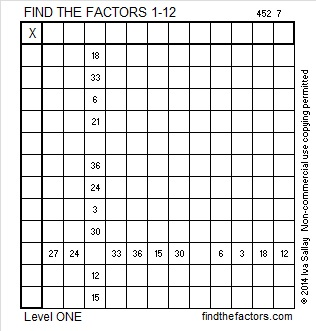Print the puzzles or type the factors on this excel file: 12 Factors 2014-12-29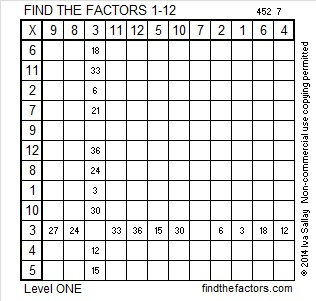# 334 and Level 6

• 334 is a composite number.
• Prime factorization: 334 = 2 x 167
• The exponents in the prime factorization are 1 and 1. Adding one to each and multiplying we get (1 + 1)(1 + 1) = 2 x 2 = 4. Therefore 334 has exactly 4 factors.
• Factors of 334: 1, 2, 167, 334
• Factor pairs: 334 = 1 x 334 or 2 x 167
• 334 has no square factors that allow its square root to be simplified. √334 ≈ 18.276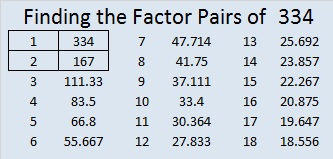Solving this Level 6 puzzle can be a little tricky: Is 1 or 2 the common factor for 6 and 8? Is 3 or 6 the common factor for 12 and 30? Is 4 or 8 the common factor for 40 and 16? In each case only one of those choices will make this puzzle work as a multiplication table? Can you figure out what those choices should be? Use logic to find the correct solution, not trial and error.Print the puzzles or type the factors on this excel file:  10 Factors 2014-12-22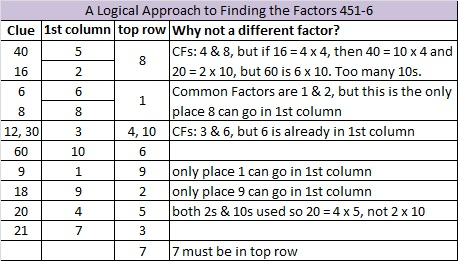# 333 and Level 5

• 333 is a composite number.
• Prime factorization: 333 = 3 x 3 x 37, which can be written 333 = 3² x 37
• The exponents in the prime factorization are 2 and 1. Adding one to each and multiplying we get (2 + 1)(1 + 1) = 3 x 2  = 6. Therefore 333 has exactly 6 factors.
• Factors of 333: 1, 3, 9, 37, 111, 333
• Factor pairs: 333 = 1 x 333, 3 x 111, or 9 x 37
• Taking the factor pair with the largest square number factor, we get √333 = (√9)(√37) = 3√37 ≈ 18.248Can the eleven clues in this puzzle help you to complete this multiplication table?Print the puzzles or type the factors on this excel file:  10 Factors 2014-12-22# 332 and a Christmas Star

• 332 is a composite number.
• Prime factorization: 332 = 2 x 2 x 83 which can be written 2² x 83
• The exponents in the prime factorization are 1 and 1. Adding one to each and multiplying we get (2 + 1)(1 + 1) = 3 x 2 = 6. Therefore 332 has exactly 6 factors.
• Factors of 332: 1, 2, 4, 83, 166, 332
• Factor pairs: 332 = 1 x 332, 2 x 166, or 4 x 83
• Taking the factor pair with the largest square number factor, we get √332 = (√4)(√83) = 2√83 ≈ 18.221Merry Christmas! This is a rather easy level 5 puzzle so I’m sharing it instead of a level 4 puzzle today.Print the puzzles or type the factors on this excel file:  10 Factors 2014-12-22

The Night Wind excitedly asked a little lamb and the whole world, “Do you see what I see?” when it saw “a star, a star, dancing in the night” after the Christ child was born. Click here to read the complete lyrics of “Do You Hear What I Hear.”# 331 and Hockey Sticks

• 331 is a prime number.
• Prime factorization: 331 is prime.
• The exponent of prime number 331 is 1. Adding 1 to that exponent we get (1 + 1) = 2. Therefore 331 has exactly 2 factors.
• Factors of 331: 1, 331
• Factor pairs: 331 = 1 x 331
• 331 has no square factors that allow its square root to be simplified. √331 ≈ 18.193How do we know that 331 is a prime number? If 331 were not a prime number, then it would be divisible by at least one prime number less than or equal to √331 ≈ 18.193. Since 331 cannot be divided evenly by 2, 3, 5, 7, 11, 13, or 17, we know that 331 is a prime number.Print the puzzles or type the factors on this excel file:  10 Factors 2014-12-22

Today’s puzzle looks like a hockey stick. Hockey sticks remind me not only of the obvious winter sport but also of the Twelve Days of Christmas and Pascal’s triangle.

Dimacs.rutgers.edu explains quite nicely how a hockey stick in Pascal’s triangle can give you the total number of gifts received after one day, two days, three days, and so on. Look at the green and red hockey stick with bold black numbers in this illustration of Pascal’s triangle:If someone gave you one partridge every day for 12 days, two turtle doves every day for 11 days, three French hens every day for 10 days, etc, etc, and etc, then you would receive 364 gifts. (364 is so easy to remember because it is one less than the number of days in a year.)

A Logical Approach to FIND THE FACTORS: Find the column or row with two clues and find their common factor. Write the corresponding factors in the factor column (1st column) and factor row (top row).  Because this is a level three puzzle, you have now written a factor at the top of the factor column. Continue to work from the top of the factor column to the bottom, finding factors and filling in the factor column and the factor row one cell at a time as you go.

# 330 Christmas Factor Trees

### Today’s Puzzle:

Can you find the factors and complete this Christmas tree multiplication table?Print the puzzles or type the factors on this excel file:  10 Factors 2014-12-22

### Factor Trees for 330:

Within these seven factor trees for 330 there are also factor trees for 6, 10, 15, 22, 30, 33, 55, 66, 110, and 165, the tops of which are all in brown. The prime factors of 330 are all in red.### Factors of 330:

• 330 is a composite number.
• Prime factorization: 330 = 2 x 3 x 5 x 11
• The exponents in the prime factorization are 1, 1, 1, and 1. Adding one to each and multiplying we get (1 + 1)(1 + 1)(1 + 1)(1 + 1) = 2 x 2 x 2 x 2 = 16. Therefore 330 has exactly 16 factors.
• Factors of 330: 1, 2, 3, 5, 6, 10, 11, 15, 22, 30, 33, 55, 66, 110, 165, 330
• Factor pairs: 330 = 1 x 330, 2 x 165, 3 x 110, 5 x 66, 6 x 55, 10 x 33, 11 x 30, or 15 x 22
• 330 has no square factors that allow its square root to be simplified. √330 ≈ 18.166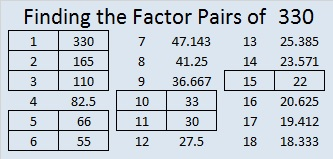### Sum-Difference Puzzle:

330 has eight factor pairs. The numbers in one of those pairs add up to 61, and the numbers in another one subtract to 61. If you can identify those factors, then you can solve this puzzle!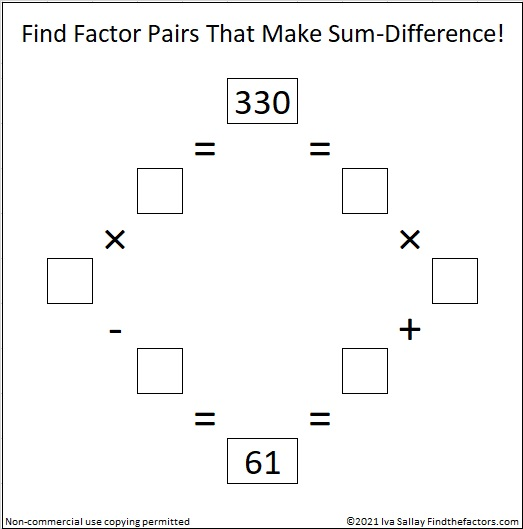### Tree Puzzle Solution: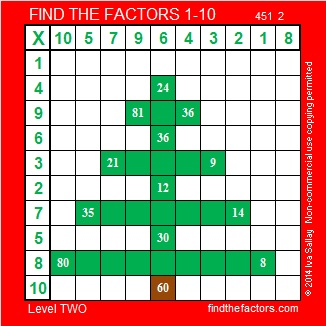# 329 and A Last Minute Gift

• 329 is a composite number.
• Prime factorization: 329 = 7 x 47
• The exponents in the prime factorization are 1 and 1. Adding one to each and multiplying we get (1 + 1)(1 + 1) = 2 x 2 = 4. Therefore 329 has exactly 4 factors.
• Factors of 329: 1, 7, 47, 329
• Factor pairs: 329 = 1 x 329 or 7 x 47
• 329 has no square factors that allow its square root to be simplified. √329 ≈ 18.138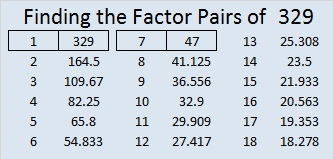Here’s a puzzle that could be a last minute gift. If there is a child in you life who has recently become familiar with the multiplication table, put this puzzle in his or her stocking! It is as easy as the puzzles get.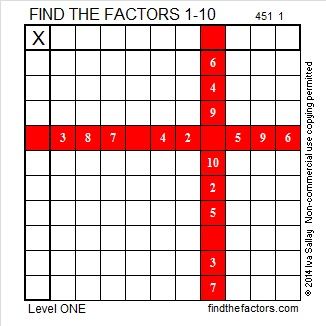Print or type on this week’s puzzles using this excel file: 10 Factors 2014-12-22Last year on Christmas Eve I offered a free last minute gift, a puzzle booklet, but I discovered that most people take care of their last minute gift-giving a couple of days sooner than that. Still this last minute gift could be sent electronically and you can’t beat the price.

I also learned that margins in excel can move so the booklet didn’t always print up as nicely as I wanted. I’m still recovering from surgery so this year I simply revised that same booklet.

I saved the booklet as Factor Holiday pdf to eliminate those printing issues.  In pdf, the lines on the puzzles became quite dark so I don’t like the way they look as much, but I’ll live with it.

The booklet is also available in Factor Holiday excel if you prefer to type your answers directly on the computer. You can try printing it off of excel as well, but I can’t guarantee what the margins will do at any given time.

Here’s a copy of the puzzle booklet’s cover with my sincere holiday greetings for all of you: